×#### Thank you for registering.

One of our academic counsellors will contact you within 1 working day.

Click to Chat

1800-1023-196

+91-120-4616500

CART 0

• 0

MY CART (5)

Use Coupon: CART20 and get 20% off on all online Study Material

ITEM
DETAILS
MRP
DISCOUNT
FINAL PRICE
Total Price: Rs.

There are no items in this cart.
Continue Shopping• Complete JEE Main/Advanced Course and Test Series
• OFFERED PRICE: Rs. 15,900
• View Details

```Chapter 13: Profits, Loss, Discount and Value Added Tax Exercise – 13.1

Question: 1

A student buys a pen for Rs 90 and sells it for Rs 100. Find his gain and gain percent.

Solution:

C.P of pen = Rs.90

S.P of pen = Rs. 100

Gain = SP - CP

= 100 - 90 = 10

Gain % = gain * 100

C.P = 10(100/90)

= 11(1/9)%

Question: 2

Rekha bought a saree for Rs.1240 and sold it for Rs. 1147. Find her loss and loss percent.

Solution:

C.P of saree = Rs. 1240

S.P of saree = Rs. 1147

Loss = CP - SP

= Rs (1240 - 1147)

= Rs. 93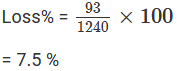Question: 3

A boy buys 9 apples for Rs. 9.60 and sells them at 11 for Rs.12. find his gain or loss percent.

Solution: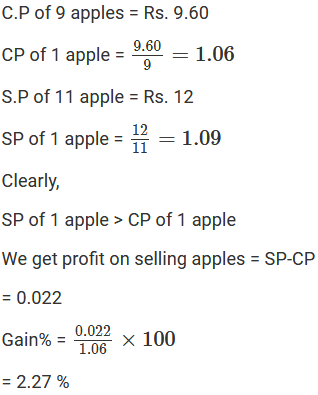Question: 4

The cost price of 10 articles is equal to the selling price of 9 articles. Find the profit percentage.

Solution:

Let the cost price of 1 article be Rs. C

Let the selling price of 1 article be Rs. S

Therefore, 10C = 9S

1 C = (9/10)S

So the cost price is less than the selling price.

Profit = S.P - C.PQuestion: 5

A retailer buys a radio for Rs.225. his overhead expense are Rs15. if he sells the radio for Rs.300, determine the profit percentage.

Solution:

Total expenses = Rs. (225 + 15) = Rs.240

S.P = Rs.300

Profit = SP - CP = Rs (300 - 240) = Rs.60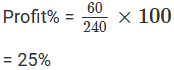Question: 6

A retailer buys a cooler for Rs.1200 and overhead expenses are on it are Rs.40. if he sells the cooler for Rs.1550, Determine the profit percentage.

Solution:

Cooler cost = Rs.1200

Total cost = Rs. (1200 + 40) = Rs.1240

S.P of the cooler = 1550

Profit = S.P - C.P

= Rs. (1550 – 1240)

= Rs. 310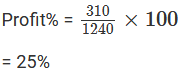Question: 7

A dealer buys a wrist watch for Rs. 225 and spends Rs.15 on its repairs. If he sells the same for Rs.300, find his profit percentage.

Solution:

A dealer buys a wrist watch for Rs.225

Money spent on repairing the watch = Rs.15

Total expenses = Rs. (225 + 15) = Rs.240

S.P = Rs.300

Profit = SP – CP = Rs (300 - 240) = Rs.60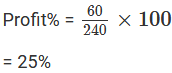Question: 8

Ramesh bought two boxes for Rs.1300. he sold one box at a profit of 20% and the other at a loss of 12%. If the selling price of both boxes.

Solution:

Let the cost price of the first box be Rs. x

Therefore, the cost of the second box will be Rs.(1300 - x)

Profit on the first box = 20%

Loss on the second box = 12%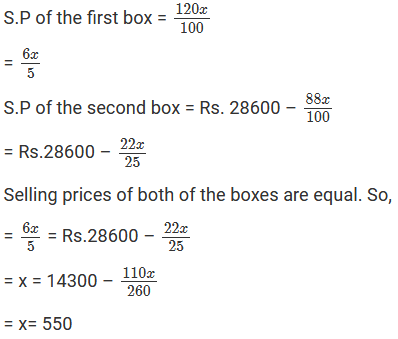The cost price of first box is Rs. 550

Cost price of the second box = Rs. (1300 - 550)

= Rs. 750

The cost prices of the 2 boxes are Rs.550 and Rs.750 respectively.

Question: 9

If the selling price of 10 pens is equal to cost price of 14 pens, find the gain percent?

Solution:

Let the cost price of one pen be Rs. C

The selling price be Rs. S

Therefore, 10S = 14C

C = (10/14)S

However, the cost price is less than the selling price.

Profit = 140 – 100

Profit % = 40%

The required profit percentage is 40%.

Question: 10

If the cost price of 18 chairs be equal to selling price of 16 chairs, find the gain or loss percent

Solution:

Let the cost price of one chair be Rs. C

Selling price be Rs. S

Therefore, 18C = 16S

However, the cost price of the chair is more than that of selling price.Question: 11

If the selling price of 18 oranges is equal to the cost price of 16 oranges, find the gain or loss percentage

Solution:

S Let the cost price of one chair be Rs. C

Selling price be Rs. S

Therefore, 18C = 16S

However, the cost price of the chair is more than that of selling price.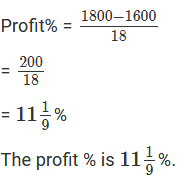Question: 12

Ravish sold his motorcycle to Vineet at a loss of 28%. Vineet spent Rs.1680 on it’s repairs and sold the motorcycle to Rahul for Rs.35910, thereby making profit of 12.5%, find the cost price of the motorcycle for Ravish.

Solution: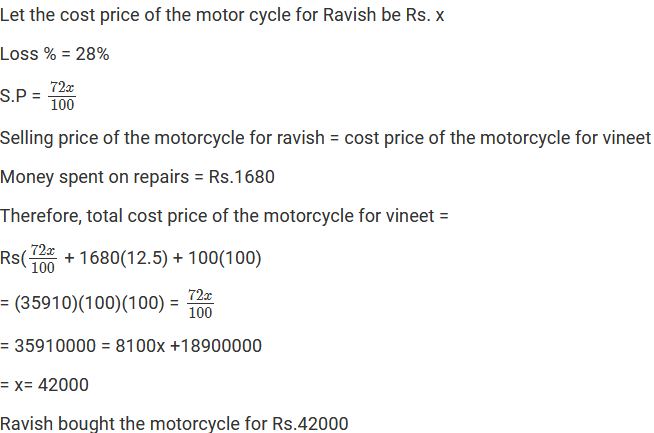Question: 13

By selling a book for Rs.258, a bookseller gains 20%. Find how much should he sell to gain 30%?

Solution:Question: 14

A defective briefcase costing Rs.800 is being sold at a loss of 8%. If the price is further reduced by 5%, find its selling price?

Solution: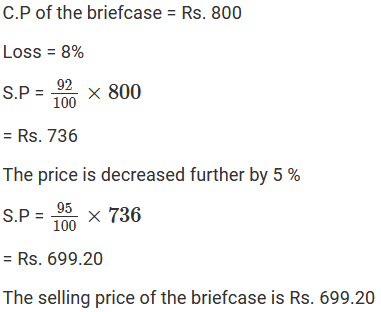Question: 15

By selling 90 ball pens for Rs160 a person loses 20%. How many ball pens should be sold at Rs.96 so as to have a profit of 20%?

Solution: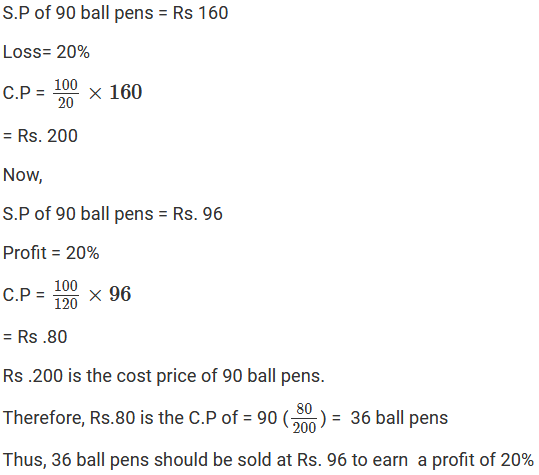Question: 16

A man sells an article at a profit of 25%. If he had bought it at a 20% less and sold it for Rs.36.75 less, he would have gained 30%. Find the cost price of the article

Solution: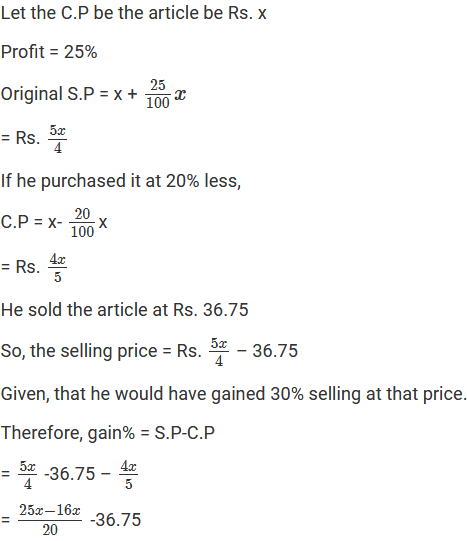Question: 17

A dishonest shopkeeper professes to sell pulses at his cost price but uses a false weight of 950 gm for each kilogram. Find his gain percentage.

Solution:Question: 18

A dealer bought two tables for Rs.3120. he sold one of them at a loss of 15% and the other at a gain of 36%. Then, he found that each table was sold for the same price. Find the cost price of each table.

Solution:

Given that the selling price is same for both the tables.

Let the C.P of 1 table be x

Then the C.P of the other table be = Rs.3120 - x

Loss on the first table = 15%

Therefore, S.P = 85 x 10

= 0.85x

Gain on the second table = 36%

136(3120 - x)

Since both the tables have the same S.P

2.21x = 4243.20

= x= 1920

The cost price of the table is Rs.1920

The cost price of the other table is Rs. (3120 - 1920) = Rs.1200

Question: 19

Mariam bought two fans Rs.3605. she sold one of them at a profit of 15% and the other one at a loss of 9 %. If Mariam obtained the same amount for each fan, find the cost price of the each of the fans.

Solution:

It is given that the S.P is same for both of the fans.

Let the C.P of the first fan be Rs. x

Therefore, C.P of the second fan be Rs. (3605 - x)

Profit on the first fan = 15%

Loss on the second fan = 6%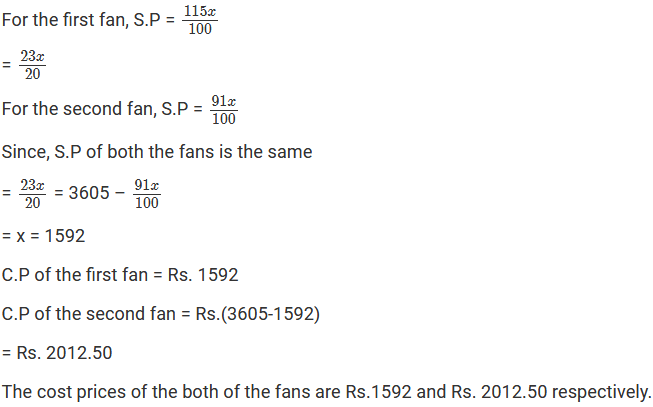Question: 20

Some toffees are bought at a rate of 11 for Rs.10 and the same number at the rate of 9 for Rs.10. if the whole lot is sold at one per toffee, find the gain or loss percent on the whole transaction.

Solution: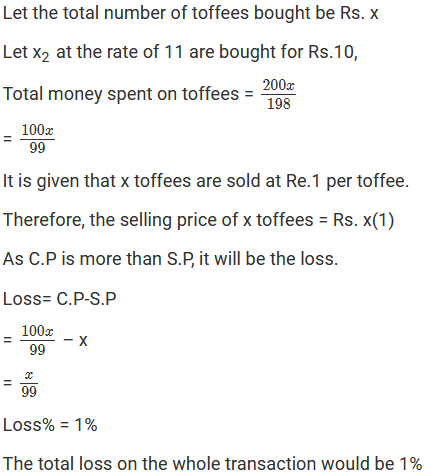Question: 21

A tricycle is sold at a gain of 16%. Had it been sold for Rs.100 more, the gain would have been 20%. Find the C.P of the tricycle.

Solution: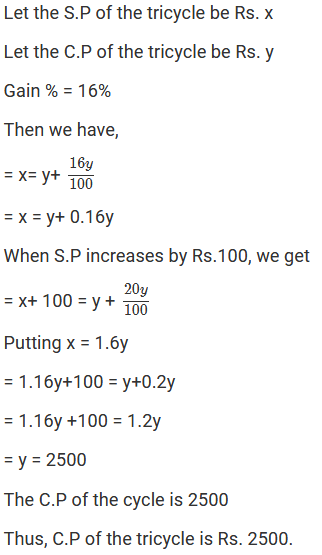Question: 22

Shabana bought 16 dozens ball pens and sold them at a loss of to S.P of 8 ball pens. Find:

(i) Her loss percent

(ii) P of 1 dozen ball pens, if she purchased these 16 dozens ball pens for Rs.576

Solution:

(i) Number of pens bought = 16(12) = 192

Let S.P of one pen be Rs. x

Therefore, S.P of 192 pens = 192x

C.P of 8 pens = Rs. 8x

It is given that S.P of 8 pens is equal to the loss on selling 192 pens.

Therefore, loss= Rs.8x

C.P of 192 pens = Rs 576

Loss = C.P – S.P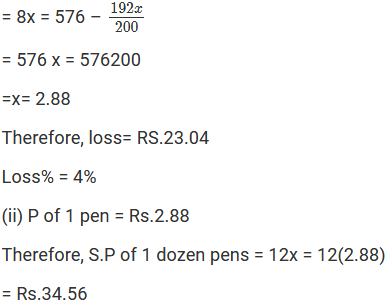Question: 23

The difference between two selling pieces of a shirt at a profit of 4 % and 5% is Rs.6. find:

(i) P of the shirt

(ii) The two selling prices of the shirt

Solution:

Let the C.P of both the shirt be RS. X

For 1 shirt profit = 4%

Profit % = Rs. 0.04x

S.P = Rs.1.04x

For 2 shirt profit = 5%

Profit% = Rs. 0.05x

S.P = Rs.1.05x

It is given that the difference between their profits is Rs.6

So, 1.05x - 1.04x = 6

= x = Rs.600

Thus, C.P = Rs.600

S.P of 1 shirt 1 = Rs.1.04x = Rs. 1.04(600) = Rs. 624

S.P of 1 shirt 2 = Rs.1.05x = Rs. 1.05(600) = Rs. 630

Question: 24

Toshiba bought 100 hens for Rs. 8000 and sold 20 of these at a gain of 5%. At what gain percent she must sell the remaining hens so as to gain 20% on the whole?

Solution: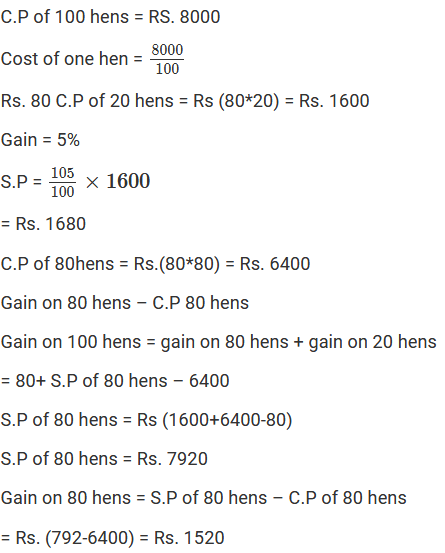```### Course Features

• 728 Video Lectures
• Revision Notes
• Previous Year Papers
• Mind Map
• Study Planner
• NCERT Solutions
• Discussion Forum
• Test paper with Video Solution